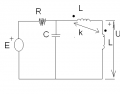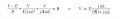# Angular frequency in AC circuit

#### cdummie

Joined Feb 6, 2015
124
So i have the circuit shown in the picture. Known values are: L=0.1mH , C=10nF and k=L12/sqrt(L1*L2) = 1. I have to find the angular frequency for which phase of generator E would be the same as the phase of voltage U.

Picture:So i know that argument of E must be equal to argument of U, since U is the voltage on inductor argument of the current through the inductor (let's call it I) is arg(I) = arg(U) - π/2.

Now, in order to find argument of E i could find the equivalent impedance of the circuit. I will start with the coupled inductors:
voltage on the inductors is U1=IjωL + IjωL12 + IjωL + IjωL12, since k=1 then L12=L so U1=Ij4ωL, so impedance of these two inductors is Z1=j4ωL, arg(Z1)=π/2, and it's in parallel with capacitor so Z2=[j4ωL*(1/jωC)]/(j(4ωL-1/(ωC))
Z2=4L/[jC(4ωL-1/(ωC))] but now i don't know what happens with argument of Z2, i mean i should somehow express argument of E but to do that, i should find the argument of Z2, but i don't know how. Is this correct approach?

#### RBR1317

Joined Nov 13, 2010
706
The solution should be quite simple, but first you need to write the node equation for the circuit. Notice that the expression for U is not necessary since the same current flows through each coil and the phase of the voltage across either coil will be the same phase as the voltage across both coils. So label the node at the center of the circuit as "V" then write the node equation for V, and solve for V. The solution for V should have the form of a ratio of jωL and a real function. When the real part is zero, the expression for V should reduce to V=E, i.e. meaning the phase of U equals the phase of E. So set the real part equal to zero and solve for ω.#### cdummie

Joined Feb 6, 2015
124
The solution should be quite simple, but first you need to write the node equation for the circuit. Notice that the expression for U is not necessary since the same current flows through each coil and the phase of the voltage across either coil will be the same phase as the voltage across both coils. So label the node at the center of the circuit as "V" then write the node equation for V, and solve for V. The solution for V should have the form of a ratio of jωL and a real function. When the real part is zero, the expression for V should reduce to V=E, i.e. meaning the phase of U equals the phase of E. So set the real part equal to zero and solve for ω.
View attachment 99866

Let's see if i understood what you said. First, phase of voltage through the two coils is the same as the phase of voltage through one coil. But, about that formula you wrote, is it sum of all currents in circuit, considering all of them are going "out" or "in" node? And that stylized R in denominator in the left expression, it represents real part, right?

#### RBR1317

Joined Nov 13, 2010
706
The usual terminology is voltage appearing 'across' and current flowing 'through' a component. The 'formula' is just how I write node equations, assuming that current exiting the node is positive. You may have a different method, but the end result should be the same. Yes, the '(R)' refers to the real part of the denominator.

#### cdummie

Joined Feb 6, 2015
124
The usual terminology is voltage appearing 'across' and current flowing 'through' a component. The 'formula' is just how I write node equations, assuming that current exiting the node is positive. You may have a different method, but the end result should be the same. Yes, the '(R)' refers to the real part of the denominator.
Ok, i did everything like you said and i got ω=5*10^5 s^-1 , but, doing it this way, it turns out that V=E which means that generator E has the same voltage as V, not only phases are the same. Yet, we have that V=IR + E (I is the current through branch with E). Which means that E cannot be equal to V unless R is zero. How come that we got E=V then?

#### RBR1317

Joined Nov 13, 2010
706
How come that we got E=V then?
What happens to the current at the condition of parallel resonance?

#### cdummie

Joined Feb 6, 2015
124
What happens to the current at the condition of parallel resonance?
Current is in phase with voltage? Is that right?

#### RBR1317

Joined Nov 13, 2010
706
Yet, we have that V=IR + E (I is the current through branch with E). Which means that E cannot be equal to V unless R is zero. How come that we got E=V then?
Is it more likely that R becomes zero or that I becomes zero when V=E?

#### cdummie

Joined Feb 6, 2015
124
Is it more likely that R becomes zero or that I becomes zero when V=E?
Well, i think R is more likely to be zero, because if I is zero then there's no current through the branch, which makes no sense since every branch must have it's current, is that right?

#### RBR1317

Joined Nov 13, 2010
706
Well, i think R is more likely to be zero
What would cause the value of R to change? Is the resistance of R a function of frequency of the generator E?
...every branch must have it's current...
What if the frequency caused an LC branch to have infinite impedance? What would the current be through an impedance of ∞? I=V/∞

Last edited:

#### cdummie

Joined Feb 6, 2015
124
What would cause the value of R to change? Is the resistance of R a function of frequency of the generator E?

What if the frequency caused an LC branch to have infinite impedance? What would the current be through an impedance of ∞? I=V/∞
Well, i think that R can't change, it is what it is, and in this example, it's value is not defined. R is not the function of the frequency of generator.

Current through impedance of ∞ is zero. How can i relate all this this to my example?

#### RBR1317

Joined Nov 13, 2010
706
Current through impedance of ∞ is zero. How can i relate all this this to my example?
Didn't you provide the answer in #8? "Yet, we have that V=IR + E (I is the current through branch with E)."

#### cdummie

Joined Feb 6, 2015
124
Didn't you provide the answer in #8? "Yet, we have that V=IR + E (I is the current through branch with E)."
Ok, so this means that I is zero or what? I am bit confused now.

#### RBR1317

Joined Nov 13, 2010
706
Ok, so this means that I is zero or what?
What it means is that at the frequency where V=E, and the phase of U is the same as the phase of E, there is no current flowing through R. You derived that result from analysis of the node equation, but could have observed directly that the impedance of the LC branch becomes infinite at parallel resonance. Have you tried to calculate how much current is flowing in L at resonance, even though there is no current flowing in R?

#### cdummie

Joined Feb 6, 2015
124
What it means is that at the frequency where V=E, and the phase of U is the same as the phase of E, there is no current flowing through R. You derived that result from analysis of the node equation, but could have observed directly that the impedance of the LC branch becomes infinite at parallel resonance. Have you tried to calculate how much current is flowing in L at resonance, even though there is no current flowing in R?
Well, i am know quite sure about that, i assume that there is no current there, since the current through the branch with generator is zero, so, no generators in circuit, no current in circuit.

#### RBR1317

Joined Nov 13, 2010
706
...so, no generators in circuit,...
The generator is still there. While no current flows through the resistor, plenty of current is flowing through L and C. But the current flowing in L and C is out of phase, cancelling each other so the resultant current is zero. The highest signal voltage across the LC tank occurs at the resonant frequency. Radio tuners work that way, usually employing a variable capacitor to change the channel frequency.